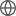Old Web
English
We present deep Chandra observations of PSO J231.6576$-$20.8335, a quasar at redshift z=6.59 with a nearby (${\sim}8$ proper kpc) companion galaxy. ALMA observed both the quasar and companion to be bright in [C II], and the system has significant extended Ly$\alpha$ emission around the quasar, suggesting that a galaxy merger is ongoing. Unlike previous studies of two similar systems, and despite observing the system with Chandra for 140 ks, we do not detect the companion in X-rays. The quasar itself is detected, but only $13.3^{+4.8}_{-3.7}$ net counts are observed. From a basic spectral analysis, the X-ray spectrum of the quasar is soft (hardness ratio of $\mathcal{HR} = -0.60_{-0.27}^{+0.17}$, power-law index of $\Gamma=2.6^{+1.0}_{-0.9}$), which results in a rest-frame X-ray luminosity comparable to other bright quasars ($L_{2-10} = 1.09^{+2.20}_{-0.70}\times 10^{45}\ \textrm{erg}\ \textrm{s}^{-1}$) despite the faint observed X-ray flux. We highlight two possible interpretations of this result: the quasar has a steep value of $\Gamma$ -- potentially related to observed ongoing Eddington accretion -- thereby pushing much of the emission out of our observed band, or the quasar has a more normal spectrum ($\Gamma{\sim}2$) but is therefore less X-ray luminous ($L_{2-10} \sim 0.6 \times 10^{45}\ \textrm{ erg}\ \textrm{ s}^{-1}$).